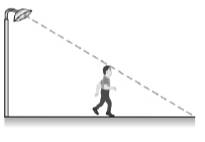Chapter 5.2, Problem 29EElementary Geometry For College St...

7th Edition
Alexander + 2 others
ISBN: 9781337614085

Solutions

Chapter
SectionElementary Geometry For College St...

7th Edition
Alexander + 2 others
ISBN: 9781337614085
Textbook Problem

In Exercises 29 to 32, use the fact that triangles are similar.Rhydian while walking away from a 10 -ft lamppost casts a shadow 6 ft long. If Rhydian is at a distance of 10 ft from the lamppost at that moment, what is Rhydian’s height?To determine

To calculate:

The Rhydian’s height when he is at a distance of 10ft from the lamppost at that moment.

Explanation

Given:

Rhydian while walking away from a 10ft lamppost casts a shadow 6ft long.

Definition Used:

Similarity between Two polygons:

Two polygons are similar if and only if when all pairs of corresponding angles are congruent and all pairs of corresponding sides are proportional.

Calculation:

Here, AB¯ represents the lamppost, DC¯ represents the person and CE¯ represents the shadow of the person.

According to the definition of segment-addition postulate, BE=BC+CE.

It is given that the shadow of the person is CE=6ft.

The distance of the lamppost and person is BC=10ft.

So,

Still sussing out bartleby?

Check out a sample textbook solution.

See a sample solution

The Solution to Your Study Problems

Bartleby provides explanations to thousands of textbook problems written by our experts, many with advanced degrees!

Get Started